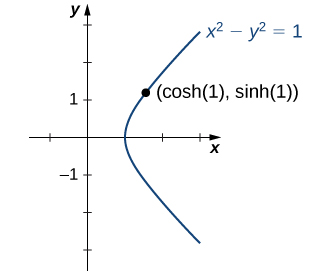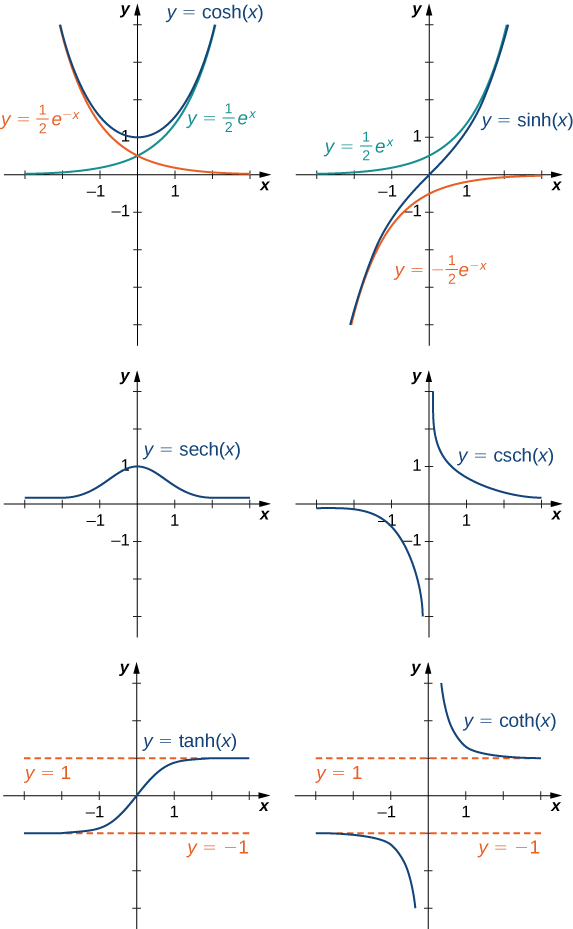# 1.5 Exponential and logarithmic functions  (Page 6/17)

 Page 6 / 17

Compare the relative severity of a magnitude $8.4$ earthquake with a magnitude $7.4$ earthquake.

The magnitude $8.4$ earthquake is roughly $10$ times as severe as the magnitude $7.4$ earthquake.

## Hyperbolic functions

The hyperbolic functions are defined in terms of certain combinations of ${e}^{x}$ and ${e}^{\text{−}x}.$ These functions arise naturally in various engineering and physics applications, including the study of water waves and vibrations of elastic membranes. Another common use for a hyperbolic function is the representation of a hanging chain or cable, also known as a catenary ( [link] ). If we introduce a coordinate system so that the low point of the chain lies along the $y$ -axis, we can describe the height of the chain in terms of a hyperbolic function. First, we define the hyperbolic functions    .The shape of a strand of silk in a spider’s web can be described in terms of a hyperbolic function. The same shape applies to a chain or cable hanging from two supports with only its own weight. (credit: “Mtpaley”, Wikimedia Commons)

## Definition

Hyperbolic cosine

$\text{cosh}\phantom{\rule{0.1em}{0ex}}x=\frac{{e}^{x}+{e}^{\text{−}x}}{2}$

Hyperbolic sine

$\text{sinh}\phantom{\rule{0.1em}{0ex}}x=\frac{{e}^{x}-{e}^{\text{−}x}}{2}$

Hyperbolic tangent

$\text{tanh}\phantom{\rule{0.1em}{0ex}}x=\frac{\text{sinh}\phantom{\rule{0.1em}{0ex}}x}{\text{cosh}\phantom{\rule{0.1em}{0ex}}x}=\frac{{e}^{x}-{e}^{\text{−}x}}{{e}^{x}+{e}^{\text{−}x}}$

Hyperbolic cosecant

$\text{csch}\phantom{\rule{0.1em}{0ex}}x=\frac{1}{\text{sinh}\phantom{\rule{0.1em}{0ex}}x}=\frac{2}{{e}^{x}-{e}^{\text{−}x}}$

Hyperbolic secant

$\text{sech}\phantom{\rule{0.1em}{0ex}}x=\frac{1}{\text{cosh}\phantom{\rule{0.1em}{0ex}}x}=\frac{2}{{e}^{x}+{e}^{\text{−}x}}$

Hyperbolic cotangent

$\text{coth}\phantom{\rule{0.1em}{0ex}}x=\frac{\text{cosh}\phantom{\rule{0.1em}{0ex}}x}{\text{sinh}\phantom{\rule{0.1em}{0ex}}x}=\frac{{e}^{x}+{e}^{\text{−}x}}{{e}^{x}-{e}^{\text{−}x}}$

The name cosh rhymes with “gosh,” whereas the name sinh is pronounced “cinch.” Tanh , sech , csch , and coth are pronounced “tanch,” “seech,” “coseech,” and “cotanch,” respectively.

Using the definition of $\text{cosh}\left(x\right)$ and principles of physics, it can be shown that the height of a hanging chain, such as the one in [link] , can be described by the function $h\left(x\right)=a\phantom{\rule{0.1em}{0ex}}\text{cosh}\left(x\text{/}a\right)+c$ for certain constants $a$ and $c.$

But why are these functions called hyperbolic functions ? To answer this question, consider the quantity ${\text{cosh}}^{2}t-{\text{sinh}}^{2}t.$ Using the definition of $\text{cosh}$ and $\text{sinh},$ we see that

${\text{cosh}}^{2}t-{\text{sinh}}^{2}t=\frac{{e}^{2t}+2+{e}^{-2t}}{4}-\frac{{e}^{2t}-2+{e}^{-2t}}{4}=1.$

This identity is the analog of the trigonometric identity ${\text{cos}}^{2}t+{\text{sin}}^{2}t=1.$ Here, given a value $t,$ the point $\left(x,y\right)=\left(\text{cosh}\phantom{\rule{0.1em}{0ex}}t,\text{sinh}\phantom{\rule{0.1em}{0ex}}t\right)$ lies on the unit hyperbola ${x}^{2}-{y}^{2}=1$ ( [link] ).The unit hyperbola cosh 2 t − sinh 2 t = 1 .

## Graphs of hyperbolic functions

To graph $\text{cosh}\phantom{\rule{0.1em}{0ex}}x$ and $\text{sinh}\phantom{\rule{0.1em}{0ex}}x,$ we make use of the fact that both functions approach $\left(1\text{/}2\right){e}^{x}$ as $x\to \infty ,$ since ${e}^{\text{−}x}\to 0$ as $x\to \infty .$ As $x\to \text{−}\infty ,\text{cosh}\phantom{\rule{0.1em}{0ex}}x$ approaches $1\text{/}2{e}^{\text{−}x},$ whereas $\text{sinh}\phantom{\rule{0.1em}{0ex}}x$ approaches $-1\text{/}2{e}^{\text{−}x}.$ Therefore, using the graphs of $1\text{/}2{e}^{x},1\text{/}2{e}^{\text{−}x},$ and $\text{−}1\text{/}2{e}^{\text{−}x}$ as guides, we graph $\text{cosh}\phantom{\rule{0.1em}{0ex}}x$ and $\text{sinh}\phantom{\rule{0.1em}{0ex}}x.$ To graph $\text{tanh}\phantom{\rule{0.1em}{0ex}}x,$ we use the fact that $\text{tanh}\left(0\right)=1,-1<\text{tanh}\left(x\right)<1$ for all $x,\text{tanh}\phantom{\rule{0.1em}{0ex}}x\to 1$ as $x\to \infty ,$ and $\text{tanh}\phantom{\rule{0.1em}{0ex}}x\to \text{−}1$ as $x\to \text{−}\infty .$ The graphs of the other three hyperbolic functions can be sketched using the graphs of $\text{cosh}\phantom{\rule{0.1em}{0ex}}x,\text{sinh}\phantom{\rule{0.1em}{0ex}}x,$ and $\text{tanh}\phantom{\rule{0.1em}{0ex}}x$ ( [link] ).The hyperbolic functions involve combinations of e x and e − x .

## Identities involving hyperbolic functions

The identity ${\text{cosh}}^{2}t-{\text{sinh}}^{2}t,$ shown in [link] , is one of several identities involving the hyperbolic functions, some of which are listed next. The first four properties follow easily from the definitions of hyperbolic sine and hyperbolic cosine. Except for some differences in signs, most of these properties are analogous to identities for trigonometric functions.

## Rule: identities involving hyperbolic functions

1. $\text{cosh}\left(\text{−}x\right)=\text{cosh}\phantom{\rule{0.1em}{0ex}}x$
2. $\text{sinh}\left(\text{−}x\right)=\text{−}\text{sinh}\phantom{\rule{0.1em}{0ex}}x$
3. $\text{cosh}\phantom{\rule{0.1em}{0ex}}x+\text{sinh}\phantom{\rule{0.1em}{0ex}}x={e}^{x}$
4. $\text{cosh}\phantom{\rule{0.1em}{0ex}}x-\text{sinh}\phantom{\rule{0.1em}{0ex}}x={e}^{\text{−}x}$
5. ${\text{cosh}}^{2}x-{\text{sinh}}^{2}x=1$
6. $1-{\text{tanh}}^{2}x={\text{sech}}^{2}x$
7. ${\text{coth}}^{2}x-1={\text{csch}}^{2}x$
8. $\text{sinh}\left(x±y\right)=\text{sinh}\phantom{\rule{0.1em}{0ex}}x\phantom{\rule{0.1em}{0ex}}\text{cosh}\phantom{\rule{0.1em}{0ex}}y±\text{cosh}\phantom{\rule{0.1em}{0ex}}x\phantom{\rule{0.1em}{0ex}}\text{sinh}\phantom{\rule{0.1em}{0ex}}y$
9. $\text{cosh}\left(x±y\right)=\text{cosh}\phantom{\rule{0.1em}{0ex}}x\phantom{\rule{0.1em}{0ex}}\text{cosh}\phantom{\rule{0.1em}{0ex}}y±\text{sinh}\phantom{\rule{0.1em}{0ex}}x\phantom{\rule{0.1em}{0ex}}\text{sinh}\phantom{\rule{0.1em}{0ex}}y$

f(x) = x-2 g(x) = 3x + 5 fog(x)? f(x)/g(x)
fog(x)= f(g(x)) = x-2 = 3x+5-2 = 3x+3 f(x)/g(x)= x-2/3x+5
diron
pweding paturo nsa calculus?
jimmy
how to use fundamental theorem to solve exponential
find the bounded area of the parabola y^2=4x and y=16x
what is absolute value means?
Chicken nuggets
Hugh
🐔
MM
🐔🦃 nuggets
MM
(mathematics) For a complex number a+bi, the principal square root of the sum of the squares of its real and imaginary parts, √a2+b2 . Denoted by | |. The absolute value |x| of a real number x is √x2 , which is equal to x if x is non-negative, and −x if x is negative.
Ismael
find integration of loge x
find the volume of a solid about the y-axis, x=0, x=1, y=0, y=7+x^3
how does this work
Can calculus give the answers as same as other methods give in basic classes while solving the numericals?
log tan (x/4+x/2)
Rohan
Rohan
y=(x^2 + 3x).(eipix)
Claudia
Ismael
A Function F(X)=Sinx+cosx is odd or even?
neither
David
Neither
Lovuyiso
f(x)=1/1+x^2 |=[-3,1]
apa itu?
fauzi
determine the area of the region enclosed by x²+y=1,2x-y+4=0
Hi
MP
Hi too
Vic
hello please anyone with calculus PDF should share
Which kind of pdf do you want bro?
Aftab
hi
Abdul
can I get calculus in pdf
Abdul
explain for me
Usman
okay I have such documents
Fitzgerald
Hamza
How to use it to slove fraction
Hello please can someone tell me the meaning of this group all about, yes I know is calculus group but yet nothing is showing up
Shodipo
You have downloaded the aplication Calculus Volume 1, tackling about lessons for (mostly) college freshmen, Calculus 1: Differential, and this group I think aims to let concerns and questions from students who want to clarify something about the subject. Well, this is what I guess so.
Jean
Im not in college but this will still help
nothing
how en where can u apply it
Migos
how can we scatch a parabola graph
Ok
Endalkachew
how can I solve differentiation?
with the help of different formulas and Rules. we use formulas according to given condition or according to questions
CALCULUS
For example any questions...
CALCULUS
v=(x,y) وu=(x,y ) ∂u/∂x* ∂x/∂u +∂v/∂x*∂x/∂v=1
log tan (x/4+x/2)
Rohan
what is the procedures in solving number 1?

#### Get Jobilize Job Search Mobile App in your pocket Now!By Michael NelsonBy Rohini AjayBy OpenStaxBy Hoy WenBy Richley CrapoBy Mary MateraBy Jazzycazz JacksonBy Mariah HauptmanBy RhodesBy OpenStax